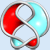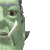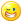## Welcome to the Second Life Forums Archive

These forums are CLOSED. Please visit the new forums HERE

# Path Maker

Seifert Surface
MathematicianJoin date: 14 Jun 2005
Posts: 912
03-09-2006 04:45
Pictures of this script in action can be seen here.

CODE
`//Path Maker by Seifert Surface//Instructions://Put this in a cube prim and reduce the x top size a little to see which direction //(x or y) is which. Rotate it so that the trapesium faces are horizontal, i.e. the //top and bottom faces lie horizontal. Make a few copies in the same horizontal //plane as the first (in general the script works as long as the prims all lie in a //plane parallel to the trapesia faces of the prims). Line the prims up roughly //with a little distance between them so  that the parallel edges of the trapesia //are the sides of a zigzag or curve. Starting off with just two prims in a rough//"V" shape might be a good example to play with first.//Very Important: number the prims (edit their descriptions) from one end of //your "snake" to the other, so the object description should read "1" on the //left, then "2", "3", etc. //Now on channel 7474, say "path", i.e. type: "/7474 path". The prims should //automagically extend and shear to make a neat path, without gaps.//Rearrange things if you want, say "path" again, rinse and repeat. There are //two other commands, again on channel 7474. "die" kills all the path prims, //and "clean" removes this script from all the path prims.//Issues: //Ok, so there will be gaps sometimes. This is usually due to the precision //allowed in the top shear and top size variables being only 1/100. Generally //some slight alteration and re-pathing will make it look better. If a prim //tries to be longer than 10m, or to have shear greater than 0.5, or less than//-0.5 (which is outside of the allowed parameters for prims) then there will //be big gaps. //The script works by extending the prim along the direction of the two parallel//edges of the trapesium faces so that those two edges meet the parallel //edges coming from its neighbouring prims. If it is not clear in which of the //two possible directions the prim should extend to meet its neighbour then //strange things can happen. This will happen if the center of a prim is nearly //in line with the parallel lines coming from one of its neighbours. Some playing//around with the script in action will help to make clear what will work and //what won't. integer Project_Number = 1035315327;  //to avoid interference with other chat channel scripts, offset by this.integer control_channel = 7474;vector pos;rotation rot;vector scl;integer listen_control;integer data_listen;float y_size = 1.0; //probably not a good idea to mess with thisfloat x_size;float x_shear;integer path_index;vector left_pos;  //parameters for the neighbouring primsrotation left_rot;float left_z;vector right_pos;rotation right_rot;float right_z;vector intersection(vector x1, vector d1, vector x2, vector d2) { //intesection of line through x1 in direction d1 with line through x2 in direction d2	//assumes these vertices and directions lie in a plane    if(llFabs(1.0 - llFabs(llVecNorm(d1) * llVecNorm(d2)) ) < 0.01) //directions parallel    {        llOwnerSay("Directions parallel warning");        return 0.5 * (x1 + x2);    }    else    {        vector n = (d1 % d2) % d2; //normal of a plane containing the line of d2        return x1 + (((n*(x2 - x1))/(n*d1)) * d1);    }} float find_x_size(vector b, vector c, vector e, vector f){    return llVecDist(e, f) / llVecDist(b, c);}float find_x_shear(vector b, vector c, vector e, vector f){    vector m1 = (b + c)/2;    vector m2 = (e + f)/2;    return ((c - b) * (m2 - m1)) / ((c - b) * (c - b));}vector get_bc(vector posn, float z_depth, rotation rotn){    return  posn - ((0.5 * (z_depth))*llRot2Up(rotn));}vector get_ef(vector posn, float z_depth, rotation rotn){    return  posn + ((0.5 * (z_depth))*llRot2Up(rotn));}work_out_params(vector b, vector c, vector e, vector f){    //  b------c    //   \    /    //    e--f    //assumes b-c is parallel to e-f                vector temp_fwd = llVecNorm(c-b);    vector temp_left = llVecNorm((e-b) % (c-b));    rot = llAxes2Rot(temp_fwd, temp_left, temp_fwd % temp_left);        float length = llVecDist(b, c);    if(length > 10.0)    {        llOwnerSay("Too much length needed!");        length = 10.0;    }    scl = llGetScale(); //not really necessary, already know this at this stage.    scl.x = length;        x_size = find_x_size(b, c, e, f);    x_shear = find_x_shear(b, c, e, f);    if(llFabs(x_shear) > 0.5)    {        llOwnerSay("Too much shear needed!");    }    pos = ((b + c) * 0.5) + ((scl.z * 0.5) * (temp_fwd % temp_left));}go_to_new_params(){    llSetPrimitiveParams([PRIM_TYPE, PRIM_TYPE_BOX, 0, <0.0, 1.0, 0.0>, 0.0, <0.0, 0.0, 0.0>, <x_size, y_size, 0.0>, <x_shear, 0.0, 0.0>,     PRIM_SIZE, scl, PRIM_ROTATION, rot, PRIM_POSITION, pos]);	//assumes we don't need to move more than 10m to get into position. If we do, "pathing" again should do it. Otherwise some kind	//of loop could be put in here.}    default{    state_entry()    {         listen_control = llListen(control_channel, "", NULL_KEY,"");    }    listen(integer channel, string name, key id, string message)    {        if(channel == control_channel)        {            if(message == "die")            {                llShout(control_channel, "die");                llDie();            }            else if(message == "clean")            {                llShout(control_channel, "clean");                llRemoveInventory(llGetScriptName());            }            else if(message == "path")            { //tell other path pieces who you are and where you are, get ready to receive their info                path_index = (integer)llGetObjectDesc();                data_listen = llListen(Project_Number + path_index, "", NULL_KEY, "");                //clear neighbour data                right_pos = ZERO_VECTOR;                right_rot = ZERO_ROTATION;                right_z = -1.0; //will be +ve if right has communicated                left_pos = ZERO_VECTOR;                left_rot = ZERO_ROTATION;                left_z = -1.0; //will be +ve if left has communicated                                vector temp = llGetScale();                string foo = (string)path_index + "|" + (string)llGetPos() + "|" + (string)llGetRot() + "|" + (string)temp.z;                llSleep(1.0); //make sure everything hears owner say "path" before sending stuff                llSay(Project_Number + path_index - 1, foo);                llSay(Project_Number + path_index + 1, foo);                llSetTimerEvent(2.0); //do stuff after waiting for data, if havent heard from both neighbours by then, I am an end of the path            }        }        else //receiving foo from others        {            list data = llParseString2List(message, ["|"], []);  //split into posn, rotns, scales            integer their_index = (integer)llList2String(data, 0);            if(their_index - path_index == 1) //to my right            {                right_pos = (vector)llList2String(data, 1);                right_rot = (rotation)llList2String(data, 2);                right_z = (float)llList2String(data, 3);            }            else if(their_index - path_index == -1) //to my left             {                left_pos = (vector)llList2String(data, 1);                left_rot = (rotation)llList2String(data, 2);                left_z = (float)llList2String(data, 3);            }           }    }    timer()    {        llSetTimerEvent(0.0);        llListenRemove(data_listen);        scl = llGetScale();        rot = llGetRot();        pos = llGetPos();        vector bc = get_bc(llGetPos(), scl.z, rot); //between b and c, the long edge        vector ef  = get_ef(llGetPos(), scl.z, rot); // the short edge        vector left_bc;        vector left_ef;        vector right_bc;        vector right_ef;        vector b;        vector e;        vector c;        vector f;                if(left_z < 0) //so we are the leftmost prim ("left" here just means numerically, according to the path_index)        {            if(right_z < 0)            {                llOwnerSay("No neighbours detected! Bad things will happen.");            }            llOwnerSay((string)path_index +" is on left end");            float which_way = 1.0;            vector right_intersect = intersection(pos, llRot2Fwd(rot), right_pos, llRot2Fwd(right_rot));            if( ( (right_intersect - pos)*llRot2Fwd(rot) ) > 0 ) //work out which direction the neighbour prim is            { //and leave the other side of the prim the same, i.e. keep the other two corners in the same place                which_way = -1.0;            }             b = bc + (which_way * (0.5 * scl.x) * llRot2Fwd(rot));            list params = llGetPrimitiveParams([PRIM_TYPE]);            vector top_shear = llList2Vector(params, 6);            vector top_size = llList2Vector(params, 5);            x_shear = top_shear.x;            x_size = top_size.x;            e = ef + scl.x * (x_shear + (which_way * x_size * 0.5)) * llRot2Fwd(rot);        }        else //we are not the leftmost prim        {            vector left_intersect = intersection(pos, llRot2Fwd(rot), left_pos, llRot2Fwd(left_rot));            if( ( (left_intersect - pos)*llRot2Fwd(rot) ) * ( (left_intersect - left_pos)*llRot2Fwd(left_rot) ) < 0)             {   //neighbour has the same idea of which way round it is, 				//based on looking at its forward direction vs (intersect - pos), if opposite signs then we are consistent                left_bc =  get_bc(left_pos, left_z, left_rot);                left_ef = get_ef(left_pos, left_z, left_rot);            }            else            {                left_bc=  get_ef(left_pos, left_z, left_rot);                left_ef = get_bc(left_pos, left_z, left_rot);            }            b = intersection(left_bc, llRot2Fwd(left_rot), bc, llRot2Fwd(rot));            e =  intersection(left_ef, llRot2Fwd(left_rot), ef, llRot2Fwd(rot));        }        if(right_z < 0)  //very similar to the stuff with the left end of the prim        {            llOwnerSay((string)path_index +" is on right end");            float which_way = 1.0;            vector left_intersect = intersection(pos, llRot2Fwd(rot), left_pos, llRot2Fwd(left_rot));            if( ( (left_intersect - pos)*llRot2Fwd(rot) ) > 0 )            {                which_way = -1.0;            }             c = bc + which_way * (0.5 * scl.x) * llRot2Fwd(rot);            list params = llGetPrimitiveParams([PRIM_TYPE]);            vector top_shear = llList2Vector(params, 6);            vector top_size = llList2Vector(params, 5);            x_shear = top_shear.x;            x_size = top_size.x;            f = ef + scl.x * (x_shear + (which_way * x_size * 0.5)) * llRot2Fwd(rot);        }        else        {            vector right_intersect = intersection(pos, llRot2Fwd(rot), right_pos, llRot2Fwd(right_rot));            if( ( (right_intersect - pos)*llRot2Fwd(rot) ) * ( (right_intersect - right_pos)*llRot2Fwd(right_rot) ) < 0)             {                   right_bc =  get_bc(right_pos, right_z, right_rot);                right_ef = get_ef(right_pos, right_z, right_rot);            }            else            {                right_bc =  get_ef(right_pos, right_z, right_rot);                right_ef = get_bc(right_pos, right_z, right_rot);            }            c = intersection(bc, llRot2Fwd(rot), right_bc, llRot2Fwd(right_rot));            f =  intersection(ef, llRot2Fwd(rot), right_ef, llRot2Fwd(right_rot));        }        if( find_x_size(b, c, e, f) > 1) //if the trapesium points the wrong way...        {  //rotate it around            vector b_temp = b;            vector c_temp = c;            b = f;            c = e;            f = b_temp;            e = c_temp;        }        if( llRot2Left(rot) * ( llVecNorm((e-b) % (c-b))  )  < 0 ) //if these corners would turn it over relative to where it is now        {  //flip it over            vector e_temp = e;            vector b_temp = b;            e = f;            b = c;            f = e_temp;            c = b_temp;        }        //if you want to see where it's trying to make a trapesium, put coloured spheres or something called         //"b", "c", "e", "f" in the prim and uncomment these.         //llRezObject("b", b, ZERO_VECTOR, ZERO_ROTATION, 0);        //llRezObject("c", c, ZERO_VECTOR, ZERO_ROTATION, 0);        //llRezObject("e", e, ZERO_VECTOR, ZERO_ROTATION, 0);        //llRezObject("f", f, ZERO_VECTOR, ZERO_ROTATION, 0);        work_out_params(b,c,e,f);        go_to_new_params();    }    state_exit()    {        llListenRemove(listen_control);    }}`
_____________________
-Seifert Surface
2G!tGLf 2nLt9cG
Nada Epoch
The LibrarianJoin date: 4 Nov 2002
Posts: 1,423
Discussion Thread
03-09-2006 20:48
/54/78/92623/1.html
_____________________
i've got nothing.